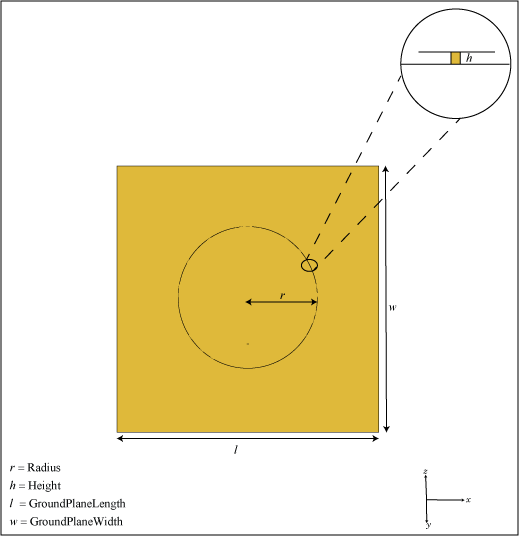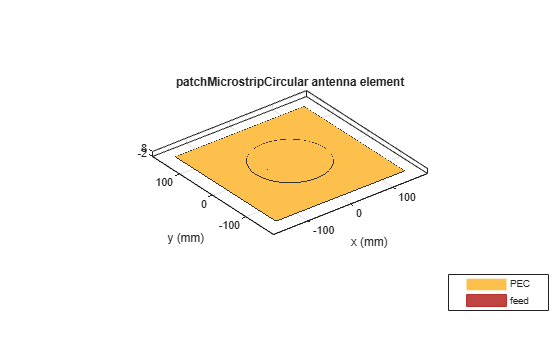# patchMicrostripCircular

Create probe-fed circular microstrip patch antenna

## Description

Use the `patchMicrostripCircular` object to create a probe-fed circular microstrip patch antenna. By default, the patch is centered at the origin with feed point along the radius and the groundplane on the xy- plane at z = 0.

Circular microstrip antennas are used as low-profile antennas in airborne and spacecraft applications. These antennas also find use in portable wireless applications because they are lightweight, low cost, and easily manufacturable.## Creation

### Syntax

``circularpatch = patchMicrostripCircular``
``circularpatch = patchMicrostripCircular(Name,Value)``

### Description

example

````circularpatch = patchMicrostripCircular` creates a probe-fed circular microstrip patch antenna. ```

example

````circularpatch = patchMicrostripCircular(Name,Value)` sets properties using one or more name-value pairs. For example, `circularpatch = patchMicrostripCircular('Radius',0.2)` creates a circular patch of radius 0.2 m. Enclose each property name in quotes.```

## Properties

expand all

Patch radius, specified as a scalar in meters. The default radius is for an operating frequency of 1 GHz.

Example: `'Radius',0.2`

Example: `circularpatch.Radius = 0.2`

Data Types: `double`

Height of patch above the ground plane along the z-axis, specified as a scalar in meters.

Example: `'Height',0.001`

Example: `circularpatch.Height = 0.001`

Data Types: `double`

Ground plane length along the x-axis, specified as a scalar in meters. Setting `'GroundPlaneLength'` to `Inf`, uses the infinite ground plane technique for antenna analysis.

Example: `'GroundPlaneLength',120e-3`

Example: ```circularpatch.GroundPlaneLength = 120e-3```

Data Types: `double`

Ground plane width along the y-axis, specified as a scalar in meters. Setting `'GroundPlaneWidth'` to `Inf`, uses the infinite ground plane technique for antenna analysis.

Example: `'GroundPlaneWidth',120e-3`

Example: ```circularpatch.GroundPlaneWidth = 120e-3```

Data Types: `double`

Type of dielectric material used as a substrate, specified as a dielectric material object. You can choose any material from the `DielectricCatalog` or use your own dielectric material. For more information, see `dielectric`. For more information on dielectric substrate meshing, see Meshing.

Note

The substrate dimensions must be lesser than the ground plane dimensions.

Example: `d = dielectric('FR4'); 'Substrate',d`

Example: `d = dielectric('FR4'); ant.Substrate = d`

Signed distance from center along length and width of ground plane, specified as a two-element real vector with each element unit in meters. Use this property to adjust the location of the patch relative to the ground plane.

Example: `'PatchCenterOffset',[0.01 0.01]`

Example: ```circularpatch.PatchCenterOffset = [0.01 0.01]```

Data Types: `double`

Signed distance from center along length and width of ground plane, specified as a two-element real vector with each element unit in meters. Use this property to adjust the location of the feedpoint relative to the ground plane and patch.

Example: `'FeedOffset',[0.01 0.01]`

Example: ```circularpatch.FeedOffset = [0.01 0.01]```

Data Types: `double`

Type of the metal used as a conductor, specified as a metal material object. You can choose any metal from the `MetalCatalog` or specify a metal of your choice. For more information, see `metal`. For more information on metal conductor meshing, see Meshing.

Example: ```m = metal('Copper'); 'Conductor',m```

Example: ```m = metal('Copper'); ant.Conductor = m```

Lumped elements added to the antenna feed, specified as a `lumpedElement` object. You can add a load anywhere on the surface of the antenna. By default, the load is at the feed. For more information, see `lumpedElement`.

Example: `'Load',lumpedElement`, where `lumpedElement` is load added to the antenna feed.

Example: `ant.Load = lumpedElement('Impedance',75)`

Tilt angle of the antenna, specified as a scalar or vector with each element unit in degrees. For more information, see Rotate Antennas and Arrays.

Example: `Tilt=90`

Example: `Tilt=[90 90]`,`TiltAxis=[0 1 0;0 1 1]` tilts the antenna at 90 degrees about the two axes defined by the vectors.

Note

The `wireStack` antenna object only accepts the dot method to change its properties.

Data Types: `double`

Tilt axis of the antenna, specified as:

• Three-element vector of Cartesian coordinates in meters. In this case, each coordinate in the vector starts at the origin and lies along the specified points on the X-, Y-, and Z-axes.

• Two points in space, each specified as three-element vectors of Cartesian coordinates. In this case, the antenna rotates around the line joining the two points in space.

• A string input describing simple rotations around one of the principal axes, 'X', 'Y', or 'Z'.

Example: `TiltAxis=[0 1 0]`

Example: `TiltAxis=[0 0 0;0 1 0]`

Example: `TiltAxis = 'Z'`

Data Types: `double`

## Object Functions

 `show` Display antenna, array structures or shapes `axialRatio` Axial ratio of antenna `beamwidth` Beamwidth of antenna `charge` Charge distribution on antenna or array surface `current` Current distribution on antenna or array surface `design` Design prototype antenna or arrays for resonance around specified frequency `efficiency` Radiation efficiency of antenna `EHfields` Electric and magnetic fields of antennas; Embedded electric and magnetic fields of antenna element in arrays `impedance` Input impedance of antenna; scan impedance of array `mesh` Mesh properties of metal, dielectric antenna, or array structure `meshconfig` Change mesh mode of antenna structure `optimize` Optimize antenna or array using SADEA optimizer `pattern` Radiation pattern and phase of antenna or array; Embedded pattern of antenna element in array `patternAzimuth` Azimuth pattern of antenna or array `patternElevation` Elevation pattern of antenna or array `rcs` Calculate and plot radar cross section (RCS) of platform, antenna, or array `returnLoss` Return loss of antenna; scan return loss of array `sparameters` Calculate S-parameter for antenna and antenna array objects `vswr` Voltage standing wave ratio of antenna

## Examples

collapse all

Create and view a default circular microstrip patch.

`cp = patchMicrostripCircular`
```cp = patchMicrostripCircular with properties: Radius: 0.0798 Height: 0.0060 Substrate: [1x1 dielectric] GroundPlaneLength: 0.3000 GroundPlaneWidth: 0.3000 PatchCenterOffset: [0 0] FeedOffset: [-0.0525 0] Conductor: [1x1 metal] Tilt: 0 TiltAxis: [1 0 0] Load: [1x1 lumpedElement] ```
`show(cp)`Create a circular patch antenna using given values. Display the antenna.

```cp = patchMicrostripCircular('Radius',0.0798,'Height',6e-3,... 'GroundPlaneLength',0.3,'GroundPlaneWidth',0.3,... 'FeedOffset',[-0.0525 0]); show(cp) ```Plot the pattern of the patch antenna at 1 GHz.

`pattern(cp,1e9);`Calculate the impedance of the antenna over a frequency span of 0.5 GHz to 1.5 GHz.

```f = linspace(0.5e9,1.5e9,61); impedance(cp,f);```## Version History

Introduced in R2017b